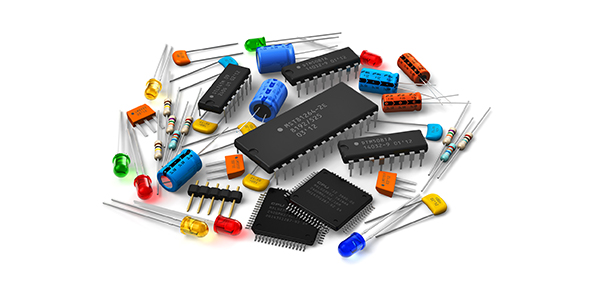# Communication Systems Revision Set 2

10 Questions | Total Attempts: 117SettingsAids in testing your knowledge of measurements of speed and error checking methods

• 1.
The number of signal events occurring each second is known as the:
• A.

Bits per second

• B.

Bandwidth

• C.

Baud rate

• D.

Modulation scheme

• 2.
A communication channel modulates waves using 256 QAM and transmits 8 million symbols each second. Approximately how long will it take to transfer 10MB?
• A.

64 seconds

• B.

8 seconds

• C.

0.125 seconds

• D.

1.25 seconds

• 3.
Which of the following includes only baseband communication links?
• A.

Ethernet, ISDN

• B.

• C.

• D.

• 4.
Which of the following is TRUE in terms of 8-bit checksums?
• A.

Approximately 99.6% of errors are detected

• B.

Approximately 99.6% of data packets will be received correctly

• C.

Approximately 99.6% of packets will not be corrupted during transmission

• D.

Approximately 99.6% of detected error can be corrected

• 5.
Protocols that include checksums include:
• A.

Ethernet and SONET

• B.

TCP and IP

• C.

ATM and IP

• D.

TCP and Ethernet

• 6.
A parity bit is added to each byte of data sent. If all data bits are reversed what will occur?
• A.

The error will always be detected

• B.

No error will ever be detected

• C.

Some errors will be detected

• D.

Most errors will be detected

• 7.
7-bit ASCII data is sent one character at a time using odd parity. The received data contains errors. Which of the following is most likely?
• A.

An odd number of bits in some bytes were corrupted

• B.

The parity bit in some bytes was corrupted

• C.

An even number of bits in some bytes were corrupted

• D.

The reciever has different port setting to the sender

• 8.
The range of frequencies a transmission channel occupies is known as its:
• A.

Symbol rate

• B.

Baud

• C.

Speed

• D.

Bandwidth

• 9.
The most significant advantage of CRCs compared to checksums is:
• A.

CRCs are used by lower OSI layer protocols than checksums

• B.

CRCs are better at detecting commonly occurring types of transmission errors

• C.

Division is a more reliable operation than addition

• D.

CRCs are usually implemented within the hardware while checksums are implemented within software

• 10.
When using parity bits, checksums and CRCs, what must occur for an error to go undetected?
• A.

The message must be corrupted such that the parity bit, checksum or CRC is unaltered

• B.

An even number of bits within the message must be corrupted

• C.

The error must be the result of hardware errors rather than software or interference errors

• D.

The message must be corrupted in such a way that it becomes some other legitimate message

Related TopicsBack to top